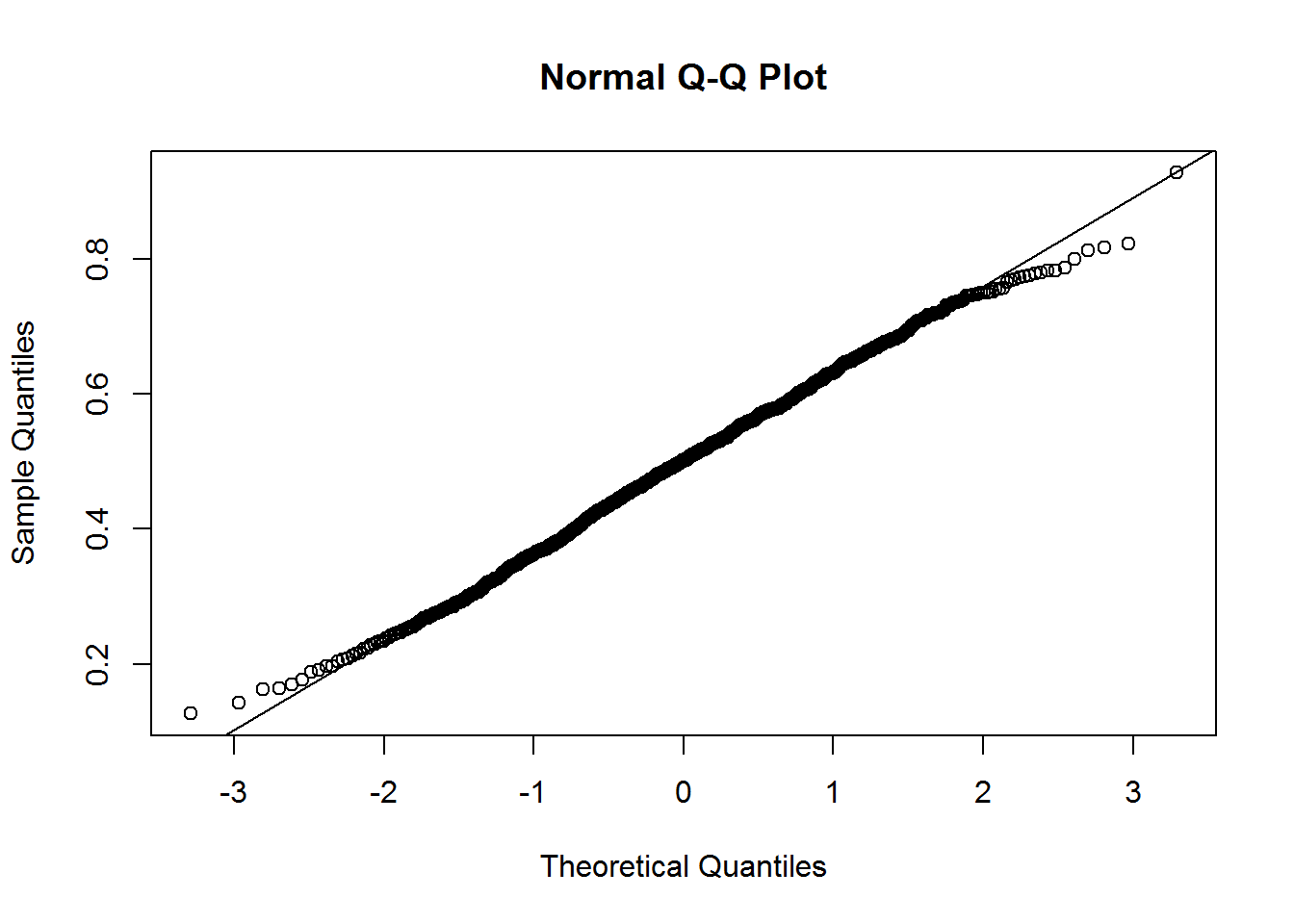#### SIMULATION OF THE CENTRAL LIMIT THEOREM:

Simulating the central limit theorem, ending up with plots side-by-side showing the bell-curve distribution of an originalNormal distribution with chosen mean and SD, which will be broad-based, as compared to the distribution of the sample means, also bell-shaped, but narrower at the base.

set.seed(2)
CentralLimit <- function(n){
means_of_samples <<-numeric()
for (i in 1:n){
means_of_samples <- c(means_of_samples,mean(rnorm(sampsize,mean,sd)))
}
samples_means <<- means_of_samples    ##The << makes the object available outside the function.
sd(samples_means)                     ## although only the sd of the samples_means will be returned
}

Now were are going to decide on a sample size, mean and standard deviation:

sampsize <- 100
mean <- 0
sd <- 1000
CentralLimit(1000)
##  105.7034
#  105.7034      Very close to theoretical: sd/sqrt(n) 1000/sqrt(100) = 100.
head(samples_means)
##   -30.69816   29.21465  142.93250  314.10070 -147.08809  252.46291
#   -30.69816   29.21465  142.93250  314.10070 -147.08809  252.46291

# Building up the population distribution using the dnorm, which will give the pdf value for every quantile fed into it:
x   <- seq(-(5 * sd),(5 * sd), length = 1000)
y   <- dnorm(x, mean, sd)

Plotting,

par(mfrow=c(1,2),oma=c(0,0,1,0))
plot(x,y, type="l",ylab="pdf",xlab="population",
frame = FALSE, col="dark blue", lwd=2, yaxt="n")
# And now the histogram of the sample means distribution:
hist(samples_means, freq = F, yaxt="n", main = "",
xlim=c(-(5 * sd(samples_means)),(5 * sd(samples_means))))

x <- seq(-(5 * sd(samples_means)),(5 * sd(samples_means)),
length=1000)
curve(dnorm(x,mean=mean(samples_means),
title("CENTRAL LIMIT THEOREM - MONTE CARLO",outer=T)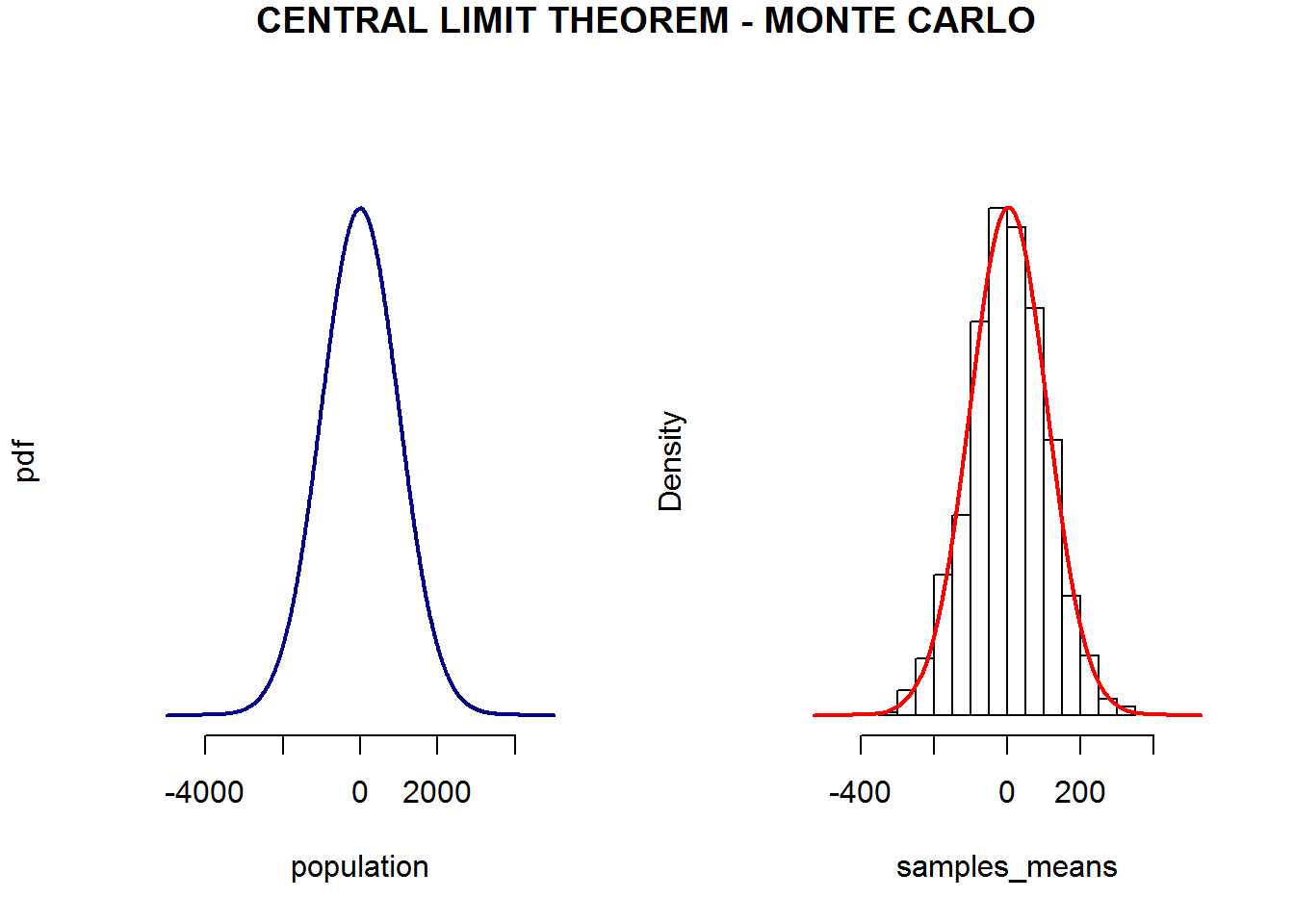For different distributions:

Distribution of sample means of 30 coin tosses with qqnorm plotting for normality and Shapiro-Wilk normality test:

tosses <- 30
samsize <- 1000
vector <- NULL
for (i in 1:1000){vector[i] = mean(rbinom(samsize,tosses,0.7))}
head(vector)
##  20.953 20.948 21.136 21.047 21.003 20.975
plot(density(vector))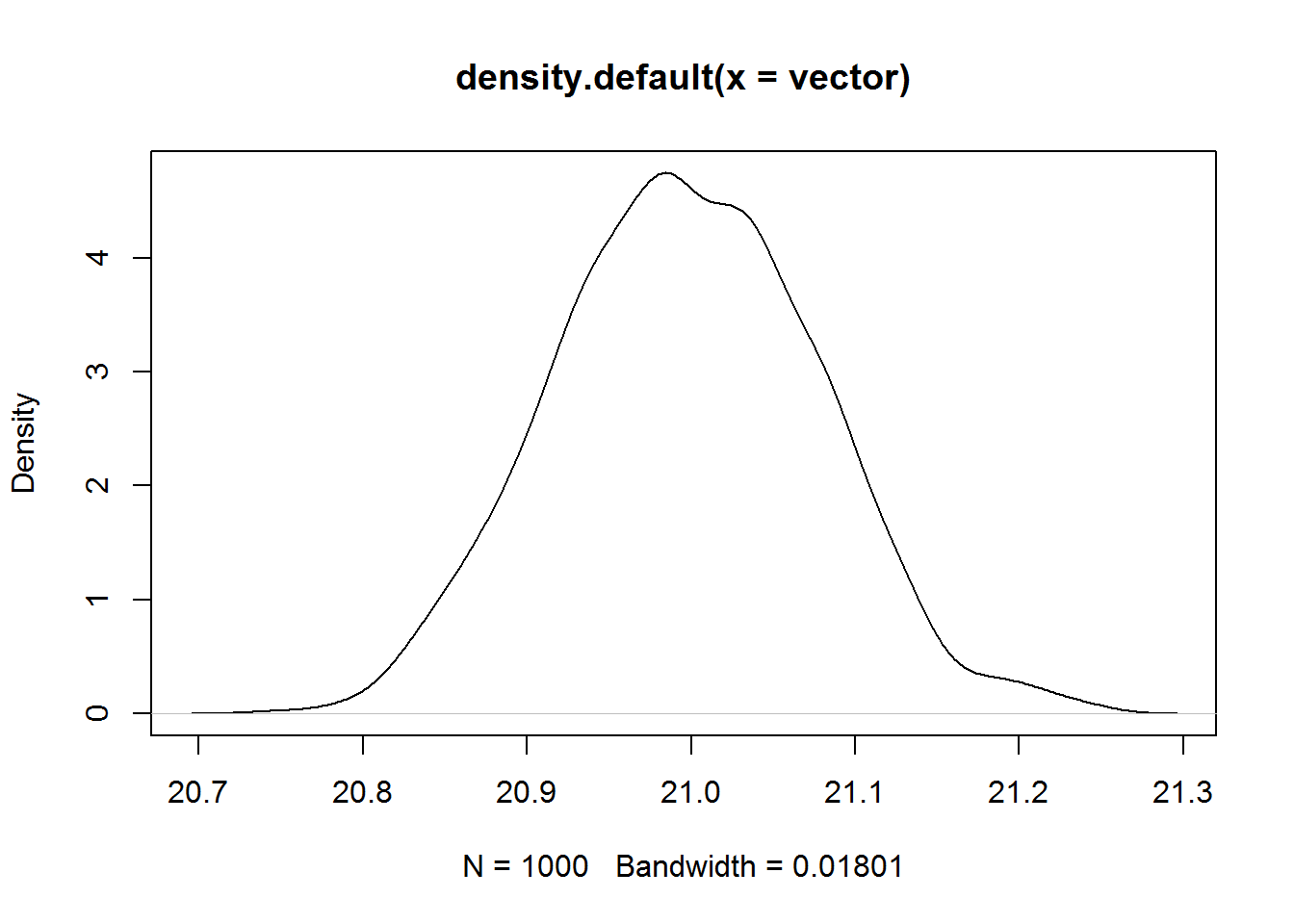shapiro.test(vector)
##
##  Shapiro-Wilk normality test
##
## data:  vector
## W = 0.99819, p-value = 0.3731
# We cannot exclude normality.
qqnorm(vector);qqline(vector)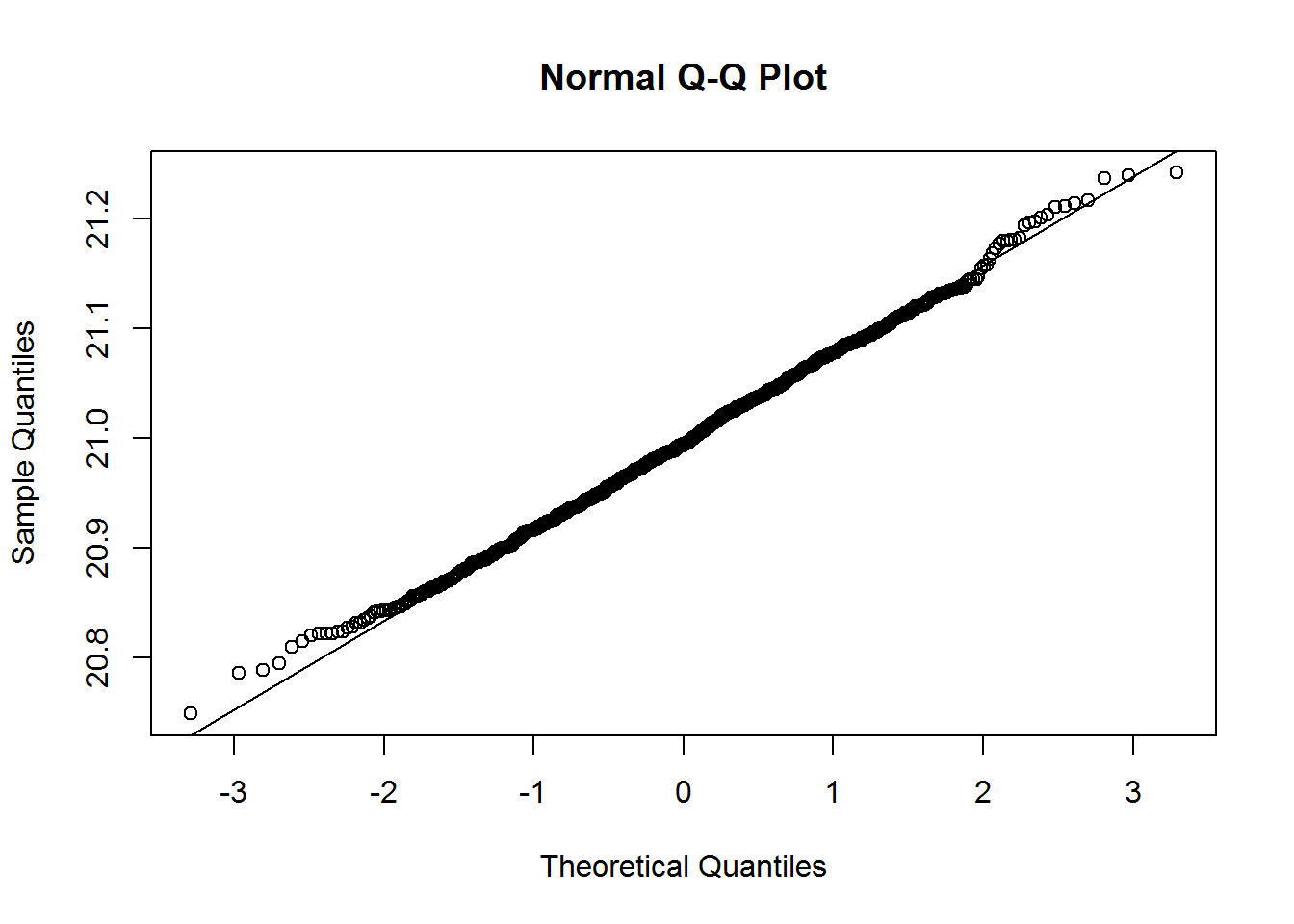Distribution of sample means of $$1000$$ samples from an Exponential with qqnorm plotting for normality and Shapiro-Wilk normality test:

vector <- NULL
for (i in 1:1000){vector[i] = mean(rexp(1000,5))}
head(vector)
##  0.2013455 0.2057055 0.1892531 0.2037769 0.1904333 0.2068772
plot(density(vector))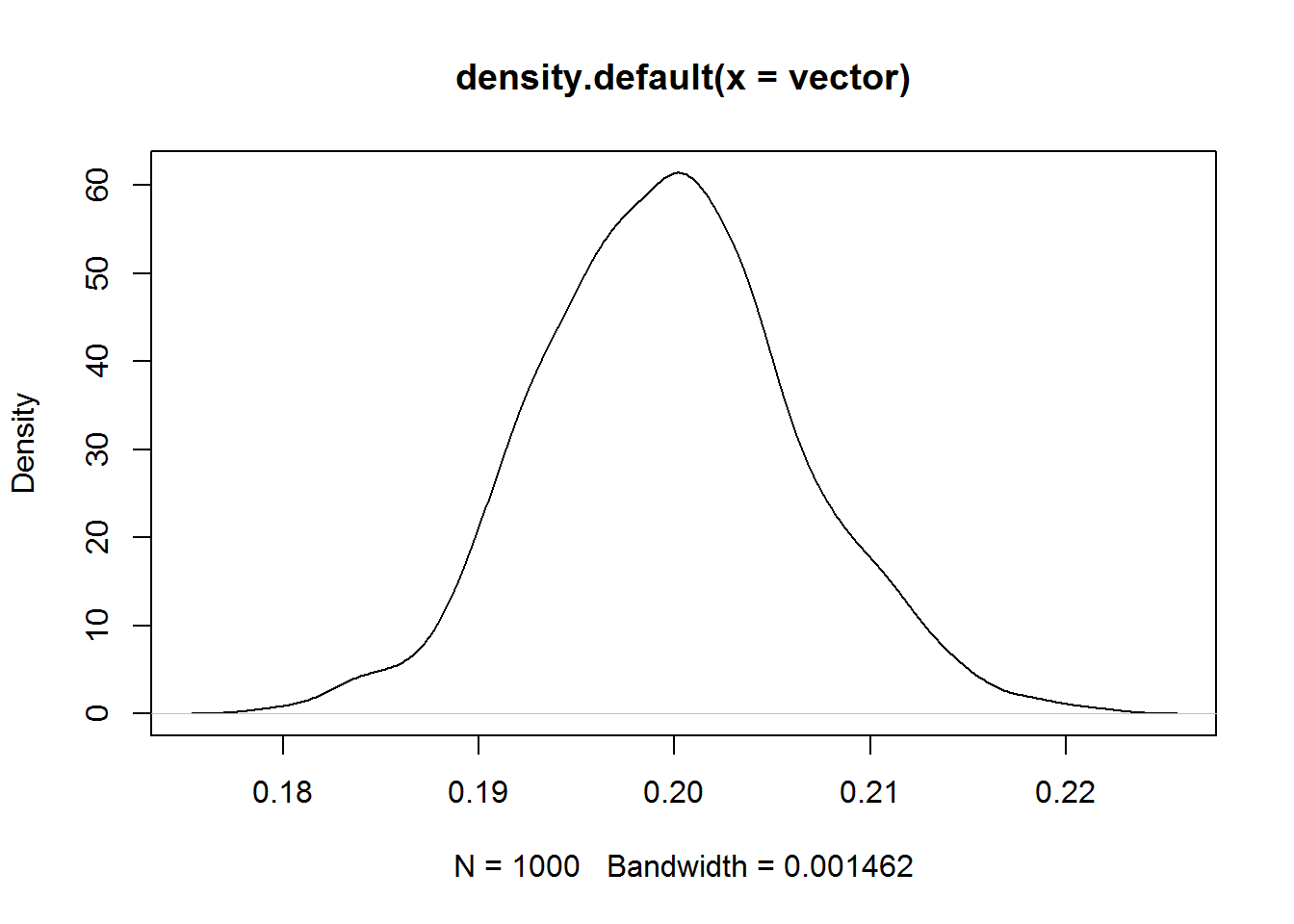shapiro.test(vector)
##
##  Shapiro-Wilk normality test
##
## data:  vector
## W = 0.99767, p-value = 0.1713
# We cannot exclude normality.
qqnorm(vector);qqline(vector)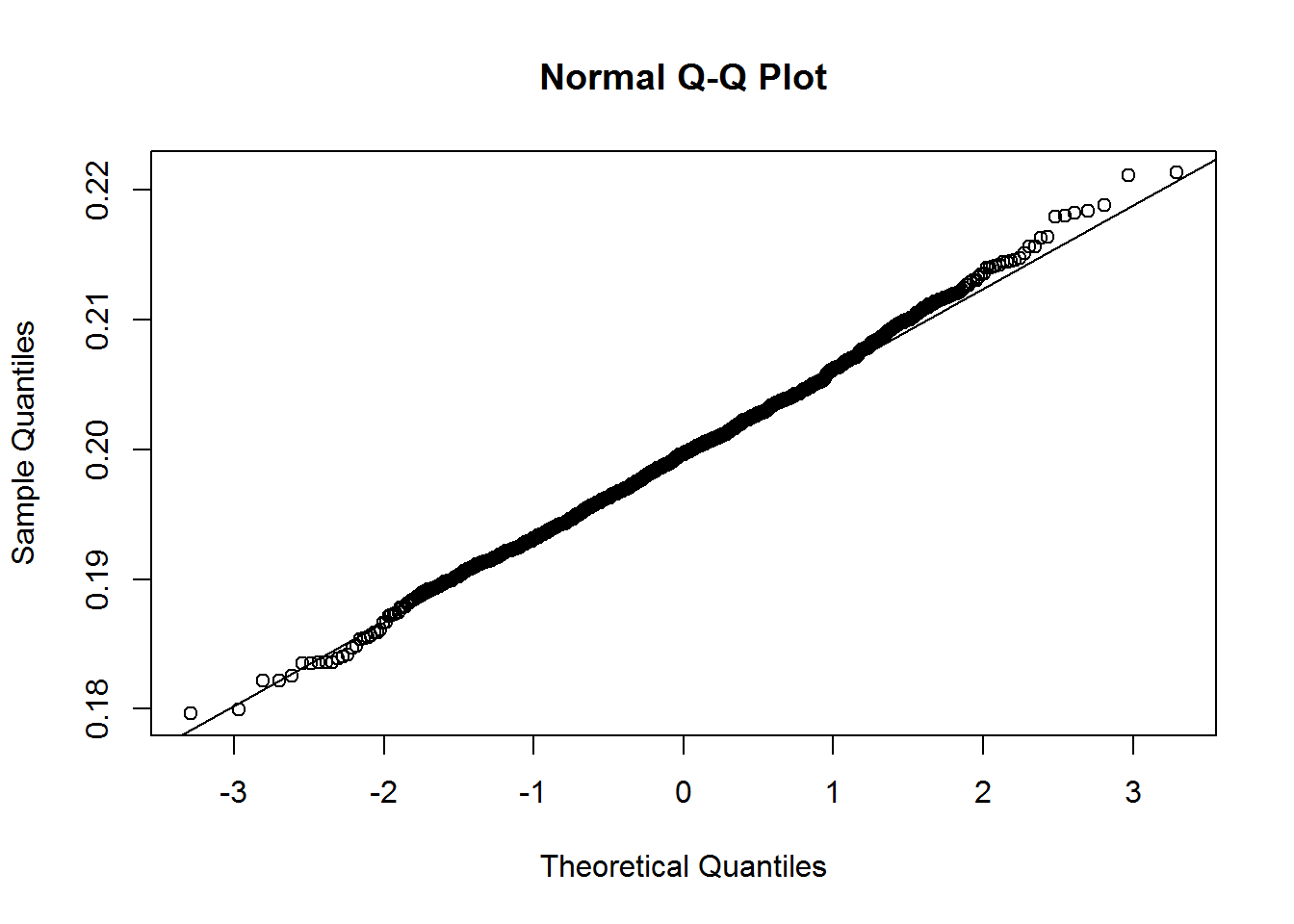Distribution of sample means of $$5$$ observation of a Uniform distr. with qqnorm plotting for normality and Shapiro-Wilk normality test:

vector1 <- NULL
for (i in 1:1000){vector1[i] = mean(runif(5))}
head(vector1)
##  0.5280128 0.6665951 0.5537420 0.2750550 0.3204791 0.6542897
plot(density(vector1))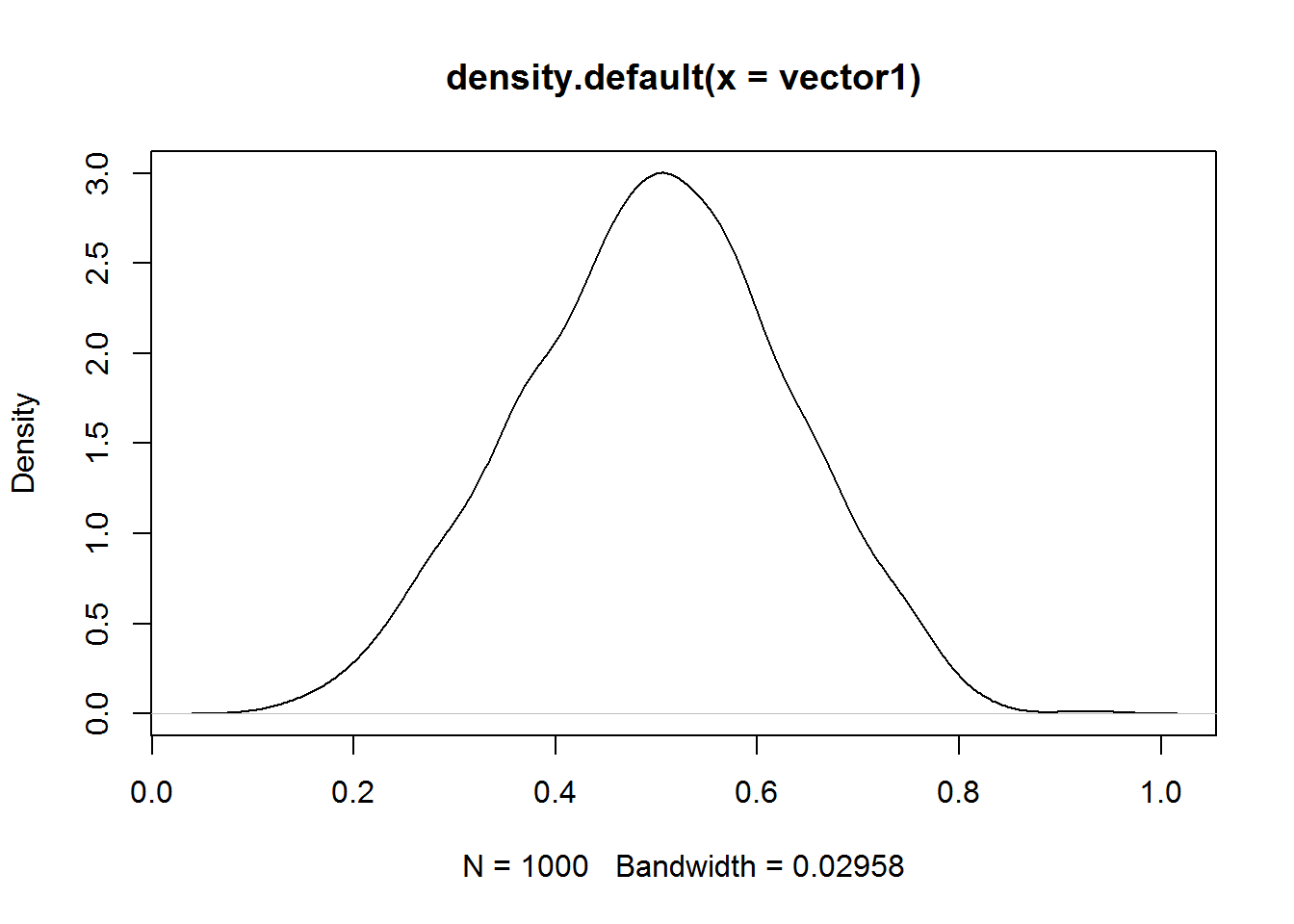shapiro.test(vector1)
##
##  Shapiro-Wilk normality test
##
## data:  vector1
## W = 0.99732, p-value = 0.09689
# We cannot exclude normality.
qqnorm(vector1);qqline(vector1)